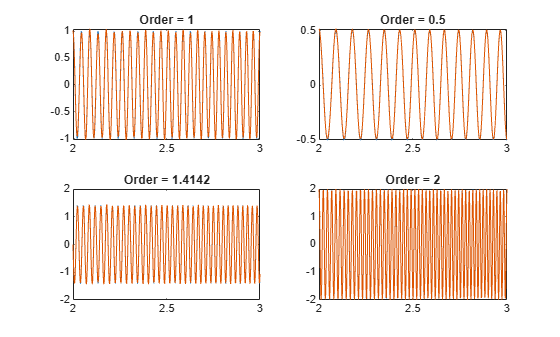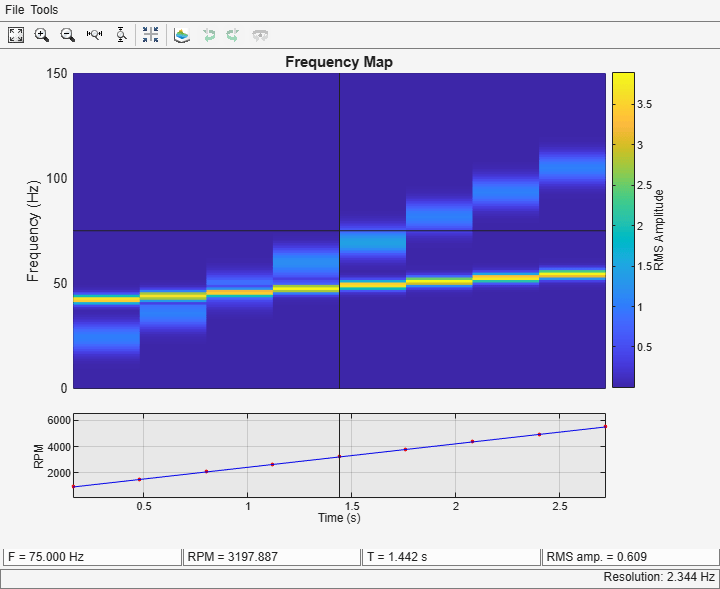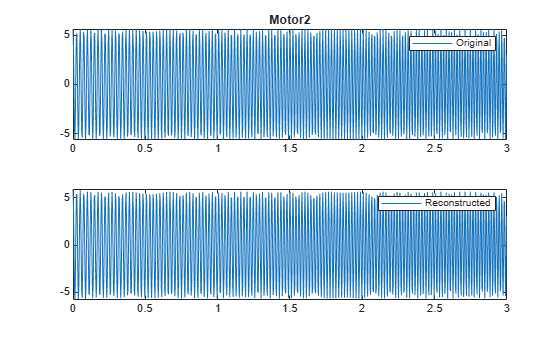# orderwaveform

Extract time-domain order waveforms from vibration signal

## Syntax

``xrec = orderwaveform(x,fs,rpm,orderlist)``
``xrec = orderwaveform(x,fs,rpm,orderlist,rpmrefidx)``
``xrec = orderwaveform(x,fs,rpm,orderlist,rpmrefidx,Name,Value)``

## Description

example

````xrec = orderwaveform(x,fs,rpm,orderlist)` returns the time-domain waveforms corresponding to a specified set of orders present in an input signal, `x`. `x` is measured at a set `rpm` of rotational speeds expressed in revolutions per minute. `fs` is the measurement sample rate in Hz. The vector `orderlist` specifies the desired orders, whose waveforms are returned in the corresponding columns of `xrec`. The function uses the Vold-Kalman filter for the computation.```
````xrec = orderwaveform(x,fs,rpm,orderlist,rpmrefidx)` returns time-domain waveforms with multiple reference RPM signals, which are stored in the columns of `rpm`. `rpmrefidx` is a vector that relates each order in `orderlist` to an RPM signal.```

example

````xrec = orderwaveform(x,fs,rpm,orderlist,rpmrefidx,Name,Value)` specifies further options for the Vold-Kalman procedure using `Name,Value` pairs.```

## Examples

collapse all

Create a simulated signal sampled at 600 Hz for 5 seconds. The system that is being tested increases its rotational speed from 10 to 40 revolutions per second (or, equivalently, from 600 to 2400 revolutions per minute) during the observation period.

```fs = 600; t1 = 5; t = 0:1/fs:t1; f0 = 10; f1 = 40; rpm = 60*linspace(f0,f1,length(t));```

The signal consists of four harmonically related chirps with orders 1, 1/2, √2, and 2. The amplitudes of the chirps are 1, 1/2, √2, and 2, respectively. To generate the chirps, use the trapezoidal rule to express the phase as the integral of the rotational speed.

```ord = [1 0.5 sqrt(2) 2]; amp = [1 0.5 sqrt(2) 2]; ph = 2*pi*cumtrapz(rpm/60)/fs; x(1,:) = amp(1)*cos(ord(1)*ph); x(2,:) = amp(2)*cos(ord(2)*ph); x(3,:) = amp(3)*cos(ord(3)*ph); x(4,:) = amp(4)*cos(ord(4)*ph); xsum = sum(x);```

Reconstruct the time-domain waveforms that compose the signal.

`xrec = orderwaveform(xsum,fs,rpm,ord);`

Visualize the results. Zoom in on a time interval occurring after the transients have decayed.

```for kj = 1:4 subplot(2,2,kj) plot(t,x(kj,:),t,xrec(:,kj)) title(['Order = ' num2str(ord(kj))]) xlim([2 3]) end```Create a simulated vibration signal consisting of two crossing orders corresponding to two different motors. The signal is sampled at 300 Hz for 3 seconds. The first motor increases its rotational speed from 10 to 100 revolutions per second (or, equivalently, from 600 to 6000 rpm) during the measurement. The second motor increases its rotational speed from 50 to 70 revolutions per second (or 3000 to 4200 rpm) during the same period.

```fs = 300; nsamp = 3*fs; rpm1 = linspace(10,100,nsamp)'*60; rpm2 = linspace(50,70,nsamp)'*60;```

The measured signal is of order 1.2 and amplitude 2√2 with respect to the first motor. With respect to the second motor, the signal is of order 0.8 and amplitude 4√2.

`x = [2 4]*sqrt(2).*cos(2*pi*cumtrapz([1.2*rpm1 0.8*rpm2]/60)/fs);`

Make the first motor excite a resonance at the middle of the frequency range.

```y = [1+1./(1+linspace(-10,10,nsamp).^4)'/2 ones(nsamp,1)].*x; x = sum(y,2);```

Visualize the orders using `rpmfreqmap`.

`rpmfreqmap(x,fs,rpm1)`Reconstruct the time-domain waveforms that compose the signal. Use the Vold-Kalman algorithm to decouple the crossing orders.

`xrec = orderwaveform(x,fs,[rpm1 rpm2],[1.2 0.8],[1 2],'Decouple',true);`

Plot the original and reconstructed waveforms.

```for kj = 1:2 figure(kj) subplot(2,1,1) plot((0:nsamp-1)/fs,y(:,kj)) legend('Original') title(['Motor ' int2str(kj)]) subplot(2,1,2) plot((0:nsamp-1)/fs,xrec(:,kj)) legend('Reconstructed') end```## Input Arguments

collapse all

Input signal, specified as a row or column vector.

Example: `cos(pi/4*(0:159))+randn(1,160)` specifies a sinusoid embedded in white Gaussian noise.

Sample rate, specified as a positive scalar expressed in Hz.

Rotational speeds, specified as a vector of positive values expressed in revolutions per minute. `rpm` must have the same length as `x`.

• If you have a tachometer pulse signal, use `tachorpm` to extract `rpm` directly.

• If you do not have a tachometer pulse signal, use `rpmtrack` to extract `rpm` from a vibration signal.

Example: `100:10:3000` specifies that a system rotates initially at 100 revolutions per minute and runs up to 3000 revolutions per minute in increments of 10.

List of orders, specified as a vector. `orderlist` must not have values larger than `fs`/(2 × max(`rpm`/60)).

Data Types: `double` | `single`

RPM column indices, specified as a vector of the same size as `orderlist`.

Data Types: `double` | `single`

### Name-Value Arguments

Specify optional comma-separated pairs of `Name,Value` arguments. `Name` is the argument name and `Value` is the corresponding value. `Name` must appear inside quotes. You can specify several name and value pair arguments in any order as `Name1,Value1,...,NameN,ValueN`.

Example: `'Decouple',true,'FilterOrder',2` extracts the specified order waveforms simultaneously and uses a second-order Vold-Kalman filter.

Vold-Kalman filter order, specified as the comma-separated pair consisting of `'FilterOrder'` and either `1` or `2`.

Data Types: `double` | `single`

Approximate half-power bandwidth, specified as the comma-separated pair consisting of `'Bandwidth'` and either a real scalar or a real vector with the same number of elements as `orderlist`. Smaller values of `'Bandwidth'` produce smooth, narrowband output. However, this output might not accurately reflect rapid changes in order amplitude.

Data Types: `double` | `single`

Mode decoupling option, specified as the comma-separated pair consisting of `'Decouple'` and a logical value. If this option is set to `true`, then `orderwaveform` extracts order waveforms simultaneously, enabling it to separate closely spaced or crossing orders.

Data Types: `logical`

Length of overlapping segments, specified as the comma-separated pair consisting of `'SegmentLength'` and an integer. If you specify a segment length, then `orderwaveform` divides the input signal into segments. It then computes the reconstructed waveforms for each segment and combines the results to produce the output. If the segments are too short, the function might not properly capture localized events such as crossing orders.

Data Types: `double` | `single`

## Output Arguments

collapse all

Reconstructed time-domain order waveforms, returned as a matrix with one waveform in each column.

 Feldbauer, Christian, and Robert Höldrich. “Realization of a Vold-Kalman Tracking Filter — A Least Squares Problem.” Proceedings of the COST G-6 Conference on Digital Audio Effects (DAFX-00). Verona, Italy, December 7–9, 2000.

 Vold, Håvard, and Jan Leuridan. “High Resolution Order Tracking at Extreme Slew Rates Using Kalman Tracking Filters.” Shock and Vibration. Vol. 2, 1995, pp. 507–515.